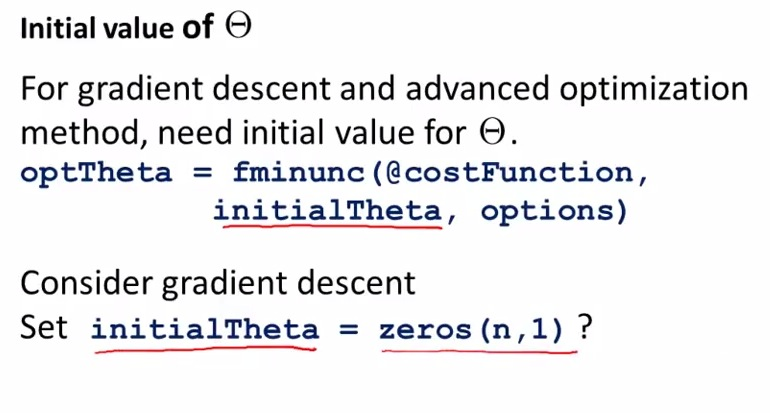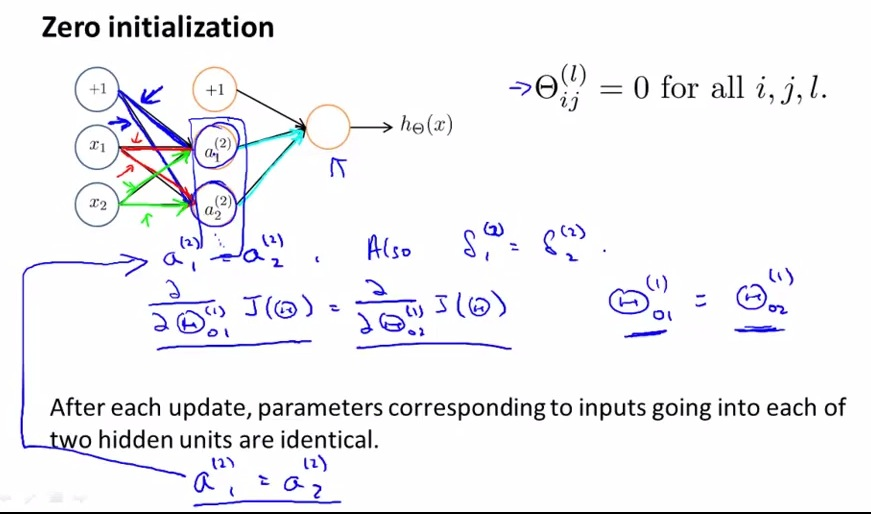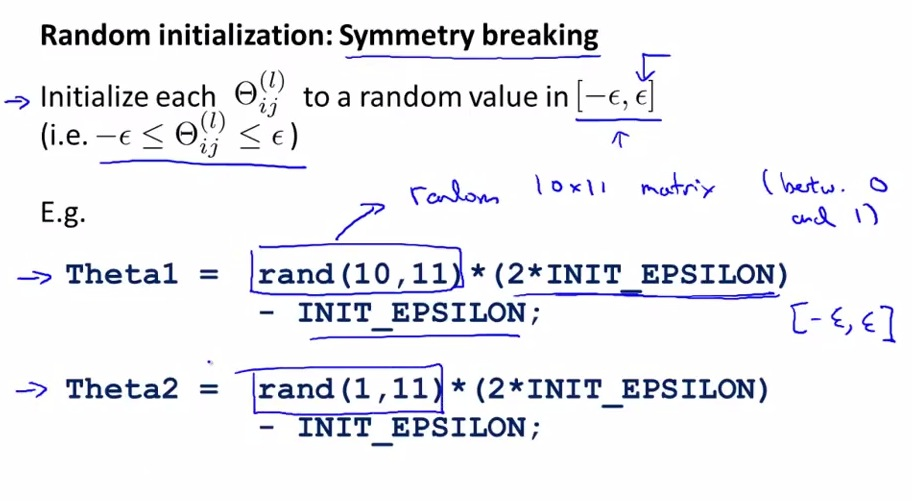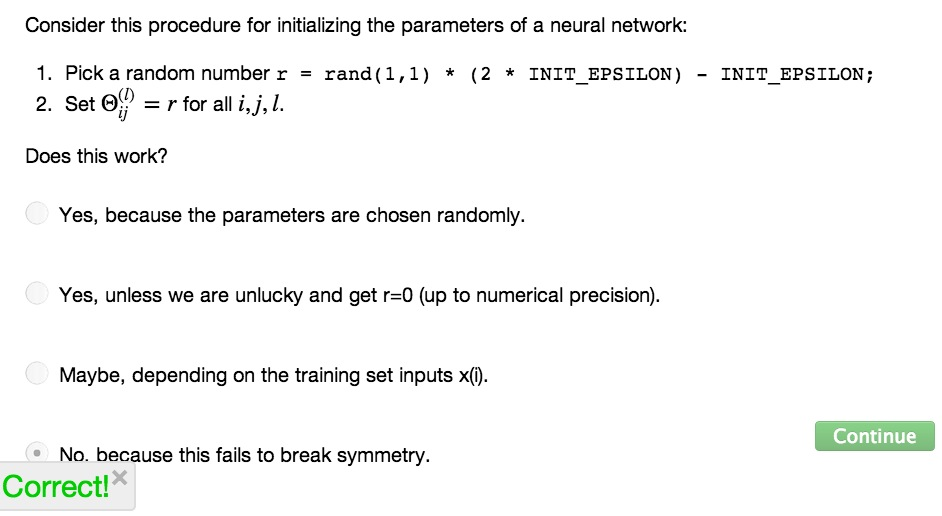• The last pieces in neural networks to be implemented• Init all theta to zero may eventually correct in logistic regression, but fail in neural networks• the result will be looping forever as the result(hidden units) is exactly is the same as input
• The problem will be solved by random initialization• the random will be the value between 0 and 1
• the epsilon is init that we manually set, different to what we know about epsilon in gradient checking• In summary, random init value close to zero
• do backprop, gradient checking, do advanced animation , to minimize cost function j(theta) using random init with symmetry breaking
• This will find a good random value of theta